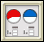Bits and Pieces I: Investigation 3: Moving Between Fractions and Decimals
 Arnold WorksiteStudent Resources Danicek WorksiteStudent Resources Grimmer WorksiteStudent Resources Homework Help Multiple-Choice Skills PracticeVocabulary Quiz Active Math Online Did You Know?Video Tutor Insert Video Links Here Pearson Video TutorCore Support Files

Decimals

Fractions

• Jamit Fractions Lessons & Games
• AAAMath Fractions, Ratios
• Illuminations Fractions
• FunSchool Action Fraction
• BBC Bitesize Fractions, Ratios
• Cool Math:
• National Library of Virtual Manipulatives
•Fraction Pieces – Work with parts and wholes to learn about fractions.Fractions - Adding – Illustrates what it means to find a common denominator and combine.Fractions - Comparing – Judge the size of fractions and plot them on a number line.Fractions - Equivalent – Illustrates relationships between equivalent fractions.Fractions - Rectangle Multiplication – Visualize and practice multiplying fractions using an area representation.
• Fraction Cafe

PercentsBits and Pieces I

(University of Michigan Overview)
Concept with Explanation
Selected Homework from ACE
In Bits and Pieces I students will investigate and make sense of rational numbers and operations with rational numbers. The investigations in this unit will help students to:

• Build an understanding of fractions, decimals, and percents and the relationships between and among these concepts and their representations;
• Develop ways to model situations involving fractions, decimals, and percents;
• Understand and use equivalent fractions to reason about situations;
• Compare and order fractions;
• Move flexibly between fraction, decimal, and percent representations;
• Use benchmarks such as 0, 1/2 , 1, and 1/4 to help estimate the size of a number or sum;
• Develop and use benchmarks that relate different forms of representations of rational numbers (for example, 50% is the same as 1/2 and 0.5);
• Use physical models and drawings to help reason about a situation;
• Look for patterns and describe how to continue the pattern;
• Use context to help reason about a situation; and
• Use estimation to understand a situation..

 Vocabulary
 Picture Vocabuary Greatest Common Factor:  The greatest number that is a factor of all numbers. Simplest Form:  The only common factor between the numerator and denominator is one. Least Common Multiple:  The least number that is a common multiple of all numbers. Equivalent Fractions:  Fractions that have the same simplest form. Power of ten:  Helps to write a decimal for a fraction whose denominator is 10, 100, 1000, ect. Benchmark:  A reference point used to determine the size of a number. Compare:  To determine which value is larger. To determine if the values are equal. Decimal:  A special form of a fraction. The denominator of this fraction is a power of 10. Order:  The way one thing follows another: In order of size Percent:  Out of 100 Fraction:  Part of a whole number. Written in the form of a numerator and denominator. Numerator:  The part of the whole being used Denominator: The whole of the fraction.  The number of equal pieces in the whole. Mixed Number:  The whole and the numerator Improper Fraction:  The numerator is larger than the denominator